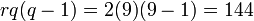# General semiaffine group:GammaA(1,9)

View a complete list of particular groups (this is a very huge list!)[SHOW MORE]

## Definition

This group is defined as the general semiaffine group of degree one over field:F9.

## Arithmetic functions

Want to compare and contrast arithmetic function values with other groups of the same order? Check out groups of order 144#Arithmetic functions

### Basic arithmetic functions

Function Value Similar groups Explanation for function value
order (number of elements, equivalently, cardinality or size of underlying set) 144 groups with same order As$\Gamma A(1,q), q = p^r, q = 9, p = 3, r = 2$:$rq(q - 1) = 2(9)(9 - 1) = 144$

### Arithmetic functions of a counting nature

Function Value Similar groups Explanation for function value
number of conjugacy classes 9 groups with same order and number of conjugacy classes | groups with same number of conjugacy classes As$\Gamma A(1,p^2), p = 3$:$p(p + 3)/2 = 3(3 + 3)/2 = 9$

## Subgroups

Important subgroups include Mathieu group:M9 and general affine group:GA(1,9).

## GAP implementation

### Group ID

This finite group has order 144 and has ID 182 among the groups of order 144 in GAP's SmallGroup library. For context, there are 197 groups of order 144. It can thus be defined using GAP's SmallGroup function as:

SmallGroup(144,182)

For instance, we can use the following assignment in GAP to create the group and name it$G$:

gap> G := SmallGroup(144,182);

Conversely, to check whether a given group$G$ is in fact the group we want, we can use GAP's IdGroup function:

IdGroup(G) = [144,182]

or just do:

IdGroup(G)

to have GAP output the group ID, that we can then compare to what we want.